# Math in Focus Grade 5 Chapter 7 Practice 2 Answer Key Equivalent Ratios

Practice the problems of Math in Focus Grade 5 Workbook Answer Key Chapter 7 Practice 2 Equivalent Ratios to score better marks in the exam.

## Math in Focus Grade 5 Chapter 7 Practice 2 Answer Key Equivalent Ratios

Write ratios to compare the two sets of items.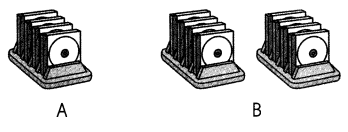Question 1.
The ratio of the number of CDs in Group A to the number of CDs in
Group B is ___ : ____
Explanation:
Given,
Number of CD’s in Group A is 4,
Number of CD’s in Group B is 8,
So,the ratio of the number of CD’s in Group A to the number of CD’s in Group B is 4 : 8

Question 2.
The ratio of the number of CD-holders in Group A to the number of CD-holders in Group B is ___ : ____
Explanation:
Given,
The number of CD – holders in Group A is 1,
The number of CD – holders in Group B is 2,
So the ratio of the number of CD – holders in Group A to the number of CD – holders in Group B is 1 : 2.

Question 3.
____ : ____ = ____ : ____ in simplest form.
Answer: 18 : 12 = 3 : 2
Explanation:
Given,
Both the numbers 18 and 12 can be divisible by 6 we get 3 : 2 to be in the simplest form.

Write ratios to compare the two sets of items.Question 4.
The ratio of the number of pencils in Group A to the number of pencils in
Group B is ___ : _____
Explanation:
In Group A there are 6 bundles of pencils each bundle has 3,
We need to multiply 6 with 3 we get 18,
In Group B there are 9 bundles of pencils each bundle has 3,
We need to multiply 9 with 3 we get 27,
So the ratio of the number of pencils in Group A to the number of pencils in Group B is 18 : 27.

Question 5.
The ratio of the number of bundles in Group A to the number of bundles in
Group B is ___ : ____
Explanation:
There are 6 bundles of pencils in Group A,
There are 9 bundles of pencils in Group B,
So the ratio of the number of bundles in Group A to the number of bundles in Group B is 6 : 9.

Question 6.
18 : 27 = 6 : 9 = ___ : ____ in simplest form.
Explanation:
Given,
Numbers 18 and 27 are divisible by 3 in the form 6 : 9,
To make it into the simplest form we need to divide by 3, we get 2 : 3.

Find the greatest common factor of each set of numbers.

Example :
4 and 6 2

Question 7.
6 and 9 _______
The greatest coomon factor of each set of numbers is 3
Explanation:
Both the numbers 6 and 9 are divisible by 3.

Quantities 8.
6 and 18 _____
Explanation:
There are four common factors of 6 and 18 that are 1,2, 3 and 6,
So the greatest common factor is 6.

Question 9.
12 and 32 ____
4
Explanation:
The greatest common factor for 12 and 32 is 4.

Complete.

Question 10.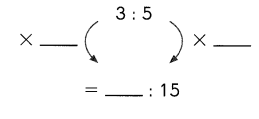Quantities 11.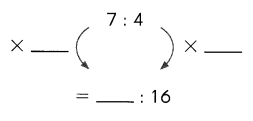Question 12.
4 : 3 = 24 : ___

Question 13.
8 : 3 = 64 : ___

Question 14.
4 : 9 = ___ : 45

Question 15.
6 : 7 = 42 : ___

Question 16.
5 : 8 = 45 : ___

Question 17.
9 : 6 = ___ : 54

Complete to express each ratio in simplest form.

Question 18.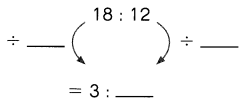Question 19.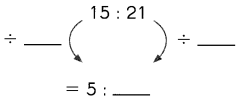Question 20.
12 : 30 = ___ : 5
Both the numbers 12 and 30 need to be divisble by 6 to get the simplest form of 2 : 5

Question 21.
14 : 28 = 1 : ___

Question 22.
60 : 45 = ___ : 3
Answer: Both the numbers 60 and 45 need to be divisble by 15 to get the simplest form of 4 : 3.

Question 23.
72 : 104 = __ : 13
Answer: Both the numbers 72 and 104 need to be divisble by 8 to get the simplest form of 9 : 13.

Question 24.
6 : 16 = __ : __
Answer: Both the numbers 6 and 16 need to be divisble by 2 to get the simplest form of 3 : 8.

Question 25.
15 : 35 = __ : ___
Answer: Both the numbers 15 and 35 need to be divisble by 5 to get the simplest form of 3 : 7.

Question 26.
4 : 48 = ___, ___
Answer: Both the numbers 4 and 48 need to be divisble by 4 to get the simplest form of 1 : 12.

Question 27.
56 : 21 = __ : ___
Answer: Both the numbers 56 and 21 need to be divisble by 7 to get the simplest form of 8 : 3.